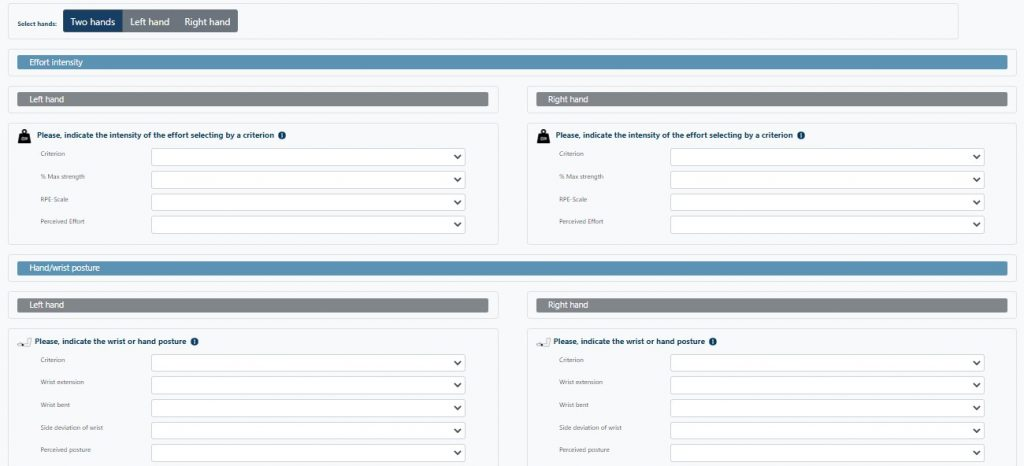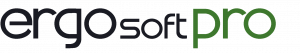# STRAIN INDEX

Originally proposed by Moore and Garg from the Department of Preventive Medicine of the Medical College of Wisconsin, United States.

It is based on identifying tasks that expose workers to a risk of developing disorders in the upper distal limbs (elbow, forearm, wrist and hand) due to repetitive motions.

Six variables are measured, which once evaluated, give rise to six multiplying factors of an equation, which result in the Strain Index (risk of disorders appearing in the upper limbs).

The variables to be measured are:

• Intensity of exertion
• Duration of exertion per work cycle
• Efforts made in a minute’s work
• Wrist deviation respect to neutral position
• Speed of work
• Duration of task per day

The variables that measure the physical exertion are the intensity of exertion and the hand/wrist posture, the other variables measure the psychological load by means of the duration of the task and the rest time.The first thing is to determine each one of the tasks carried out by the worker and the duration of the work cycles. Once the tasks to be assessed have been selected, each one of them is observed, giving values to the six variables. Once evaluated, the multiplying factors of the equation will be calculated for each task according to the method. The Strain Index will be the result of the product.

How do we interpret the Strain Index?

• Values of 3 or less indicate that the task is probably safe.
• Score of 7 or more indicate that the task is probably dangerous.### Use it to assess ergonomic risk factors in your work environment

from 149 EUR / year